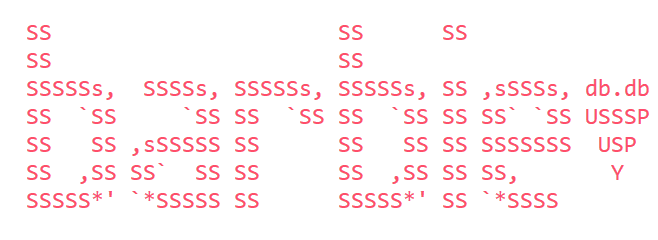Articles 16
Tags 19PROLOG - Part 3

Logic will get you from A to B. Imagination will take you everywhere.

More theory …

After last post theoretical questions were open and the time for the writing was out. I will try to answer some of them now =)

First order logic and Unification

First-order logic (FOL) or also called predicate logic has one or more arguments for each predicate and utilizes quantifiers (“for all” and “at least one”). First-order logic is simple but enough to formalize all classic mathematics. A first-order theorem, for example, consists in a set of axioms and sentences that we can reduce from them.

PROLOG is based on FOL and for that, in PROLOG one has the first-order unification algorithm implicit. Unification in logic is nothing more than an algorithmic process to solve systems of symbolic expressions. The solution of an unification problem is like a map of symbolic values for each variable in the system.

PROLOG’s unification algorithm computes a set, which is complete and minimal. This is how PROLOG binds the value of variables and you can also see it as attribution. Important points are:

• In the traditional PROLOG, the variable X without instantiation (this means, before any unification) can be unified to an atom, a term or another no instantiated variable. (In the modern PROLOG, the FOL logic check applies - a variable can not be unified with another term, which contains this same variable).
• An atom in PROLOG can be unified only with the same atom.
• Similarly, a term can only be unified with another term, if and only if, the symbols and the arity of the functions they occur are identical and if the parameters can be unified. (This is recursive!)

As PROLOG is a declarative language, the order of the unifications are not important (mostly!).

Back to the family code

Important to remember while coding in PROLOG:

• relation’s names start with small letters,
• variables start always with capital letters or underscore,
• once a variable is instantiated, it is not possible to change its object,
• all facts should end with a dot “.”.

Remember our last post query?

?- parent(isaac,X).
X = esau ;
X = jacob.

?-

Let’s understand what happens in the parent(isaac,X).: at first, the variable X is not instantiated, so PROLOG will look for a value in the knowledge base that makes our query true. For that, PROLOG will seek a fact which has the same predicate, the same arity and then it will check, if the first argument is “isaac”.

Moar technical concepts

To build more complex logical questions we can use conjunctions (AND) and disjunctions (OR) to connect the facts. In case of conjunction, we connect them with comma and in case of disjunction with semi colon.

Let’s see how it works. We query now using the conjunction operator parent(sarah,X), parent(abe,X). and the result is:

?- parent(sarah,X), parent(abe,X).
X = isaac ;
false.

?-

This means, we ask PROLOG who is child from Sarah and Abe. As we have an instance, PROLOG will answer “Isaac”. If we want to know, if Sarah and Abe have another child, we can use “;” and this time PROLOG returns “false”.